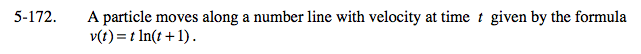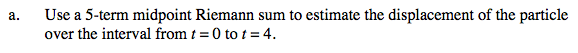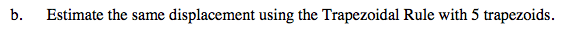### Home > CALC > Chapter 5 > Lesson 5.5.2 > Problem5-172

5-172.
1. A particle moves along a number line with velocity at time t given by the formula v(t) = t ln(t + 1). Homework Help ✎

1. Use a 5-term midpoint Riemann sum to estimate the displacement of the particle over the interval from t = 0 to t = 4.

2. Estimate the same displacement using the Trapezoidal Rule with 5 trapezoids.General setup for a midpoint Riemann sum:General setup for Trapezoidal sum on [a, b]: